## Tool Used: BeamPROP

Magneto-optic effects are phenomena in which the optical properties of a material are altered by the presence of a quasi-static magnetic field (either externally applied, or due to magnetization of the material itself). The first discovered magneto-optical effect, now known as the Faraday Effect, occurs when linearly polarized light propagates through a material that is exposed to a magnetic field aligned parallel to the direction of light propagation. Under these conditions, the plane of polarization is seen to rotate; this rotation is referred to as Faraday Rotation.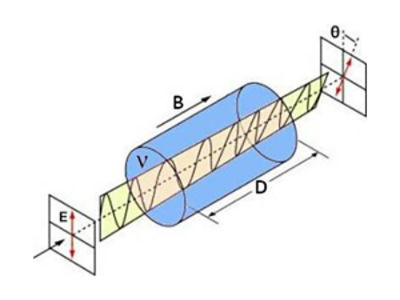Figure 1: Polarization rotation due to the Faraday effect

The strength of the polarization rotation due to the Faraday Effect is defined according to the empirical relationship: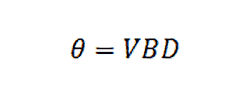Here, V is the Verdet constant of the material, D the thickness of the sample, and B the strength of the magnetic field. For most transparent materials, V is extremely small and is both temperature and wavelength dependent.

The permittivity tensor is the traditional starting point for deriving the Faraday Effect (although magneto-optic effects could be equivalently be derived from the permeability tensor). To describe the Faraday Effect, the dielectric permittivity is treated as a non-diagonal tensor taking the form (for propagation in the z-direction):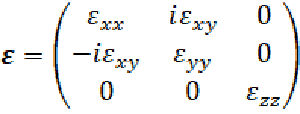Here, the direction of the applied magnetic field (or magnetization) has been taken as the z-axis. The diagonal elements represent the optical properties of the material in the absence of magneto-optic effects. The off-diagonal element Ԑxy is the origin of the Faraday Effect: it can be shown that a nonzero Ԑxy  creates a phase lag between the left-hand and right-hand circularly polarized components of linearly polarized light, resulting in rotation of the polarization plane .

One of the most distinguishing and important properties of Faraday Rotation is its non-reciprocal behavior; if light makes two opposite passes through a magneto-optic material, the Faraday Rotation does not cancel (as polarization rotation due to optical activity would) but rather doubles. This non-reciprocality allows the Faraday Effect to be used as the operating principle behind optical isolators and optical circulators, essential components in optical telecommunications and other laser applications.

## Faraday rotation in a straight fiber

To simulate Faraday Rotation, we utilize the full-vectorial, anisotropic simulation capabilities of RSoft’s BeamPROP BPM-based simulation tool. BeamPROP is particularly well-suited for modeling Faraday Rotation since, as the Faraday Effect is usually rather weak, large propagation distances are typically required. For this example, we will model Faraday Rotation in a simple straight fiber, where the material parameters of the fiber are defined by the epsilon tensor shown in Figure 2.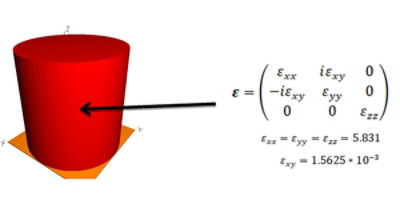Figure 2: (left) Straight fiber structure, radius=1um
(right) Epsilon tensor defining the fiber material parameters

In order to measure the polarization rotation, two pathway monitors are utilized to measure the power within the fiber core. One monitor is defined to measure the Ex component of the total field, the other monitor measures Ey. Together, these monitors allow measurements to be made that show the power rotating between the two polarizations.

The launch field for this example has been set to the fundamental fiber mode for this structure at a wavelength of 813 nm.  The initial polarization angle is set to 45°, so that both Ex and Ey are equally excited. The BeamPROP simulation results of the fiber structure depicted in Figure 2 are shown in Figure 3.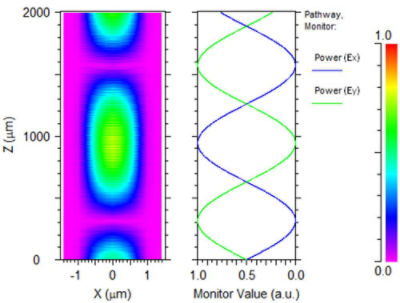Figure 3: Simulated results of rotator device. (left) Contour map of Ex amplitude
(right) Pathway monitor results for Ex and Ey

The pathway monitor plot in Figure 3. clearly shows the power of the system coupling between Ex and Ey.  For a 180-degree polarization rotation, the characteristic length for this structure is therefore about 629 μm.

In summary, BeamPROP provides a powerful tool for simulating Faraday Rotation. The results presented in this article for a straight fiber can be easily extended to more complicated situations, giving the user a versatile tool to study a wide variety of applications in optical communications, sensor applications, laser applications, and more.

## References:

 Nassau, Kurt. "Handbook of laser science and technology, supplement 2: optical materials. CRC Press, Boca Raton, FL, 1995, 833 pages, (1996).

 Bennemann, Karl Heinz. Non-linear optics in metals. No. 98. Oxford University Press, 1998.